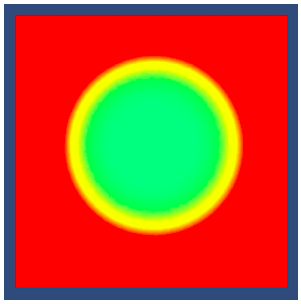# 开启HDR时，半透明渲染画面异常的解决方案

## 一、举例说明

``````// Blend 状态设置
Blend SrcAlpha OneMinusSrcAlpha

// 颜色输出
fixed4 col = 2.0f * i.color * tex2D(_MainTex, i.texcoord);
return col;
``````## 二、分析说明

``````fixed4 col = 2.0f * i.color * tex2D(_MainTex, i.texcoord);
``````

``````Blend SrcAlpha OneMinusSrcAlpha
``````

``````final color = SrcAlpha * SrcColor + (1 - SrcAlpha) * DestColor
``````

``````SrcAlpha = 2
SrcColor = fixed3(2,0,0)
DestColor = 背景色 <= fixed3(1,1,1)
``````

``````SrcAlpha = 2
SrcColor = fixed4(2,2,0)
// 上文中说明了 R 通道是一个介于 3 到 4 之间的一个值
// GB 通道是两个负值，值的大小和背景色有关
DestColor = fixed3(3.5,-g,-b)
``````

## 三、验证并修复

``````fixed4 col = i.color * tex2D(_MainTex, i.texcoord);
col.rgb *= 2.0f;
return col;
``````